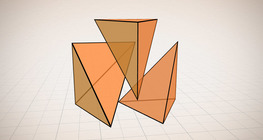Quantity: 0

Total: 0,00

# Euler's polyhedron formula

### Euler's polyhedron formula

The theorem formulated by Leonhard Euler describes one of the basic properties of convex polyhedra.

Mathematics

Keywords

Leonhard Euler, edge, vertex, face, theorem, conjecture, proof, tetrahedron, polyhedron, geometry, mathematics, mathematician

### Related items#### Császár polyhedron

The Császár polyhedron is a nonconvex polyhedron with 14 triangular faces.#### Euclid of Alexandria

The Ancient Greek scientist, Euclid of Alexandria, is often referred to as the 'father of geometry'.#### Grouping of solids 1

This animation demonstrates various groups of solids through examples.#### Grouping of solids 2

This animation demonstrates various groups of solids through examples.#### Grouping of solids 3

This animation demonstrates various groups of solids through examples.#### Grouping of solids 4

This animation demonstrates various groups of solids through examples.#### Platonic solids

This animation demonstrates the five regular three-dimensional (or Platonic) solids, the best known of which is the cube.#### Regular square pyramid

A regular square pyramid is a right pyramid with a square base and four triangular faces.#### Volume of a tetrahedron

To calculate the volume of a tetrahedron we start by calculating the volume of a prism.